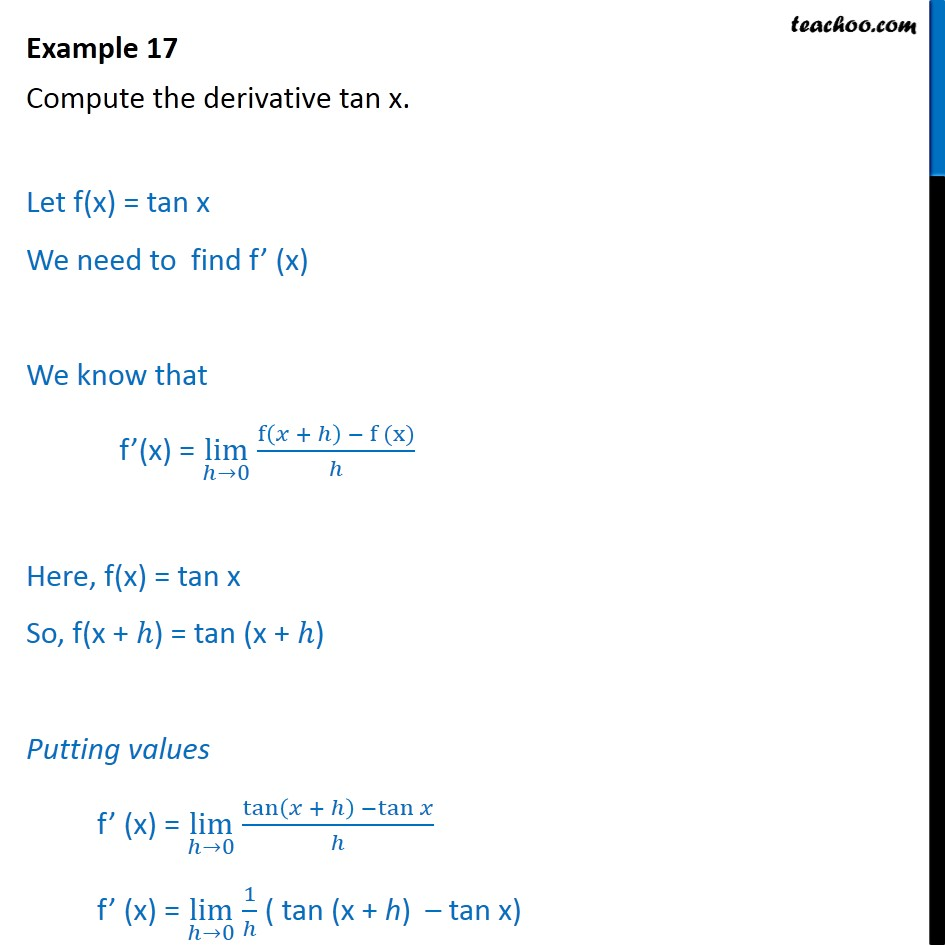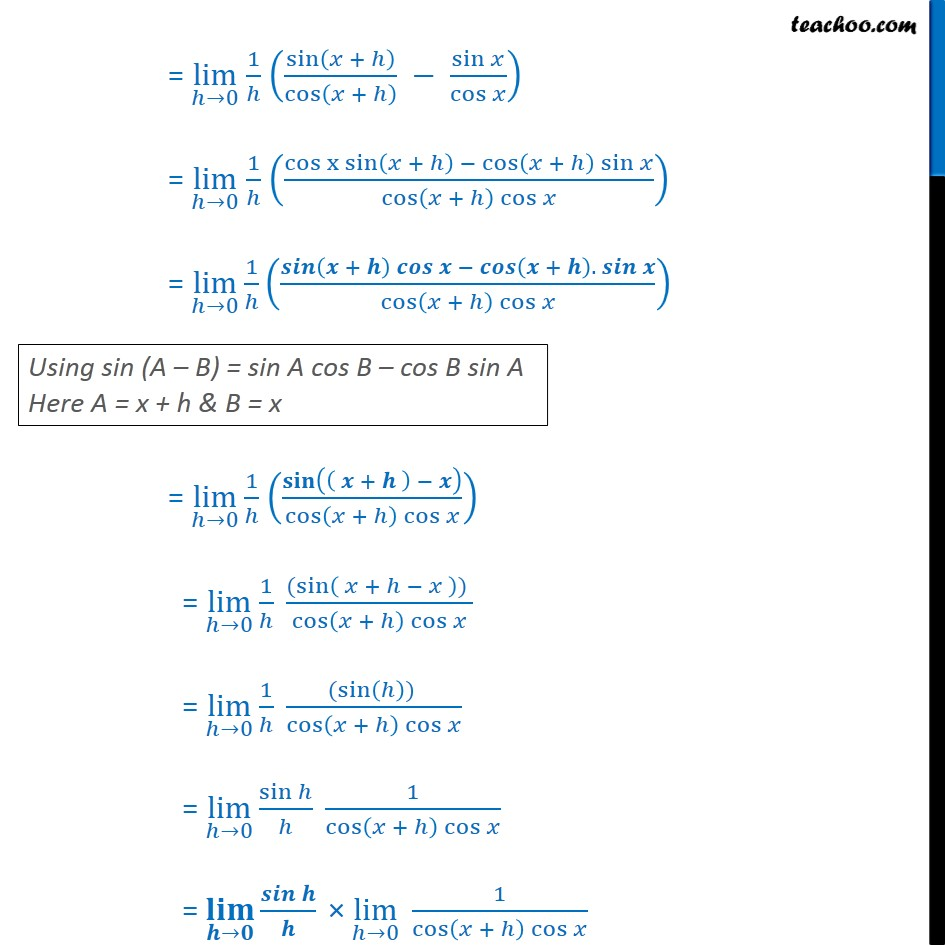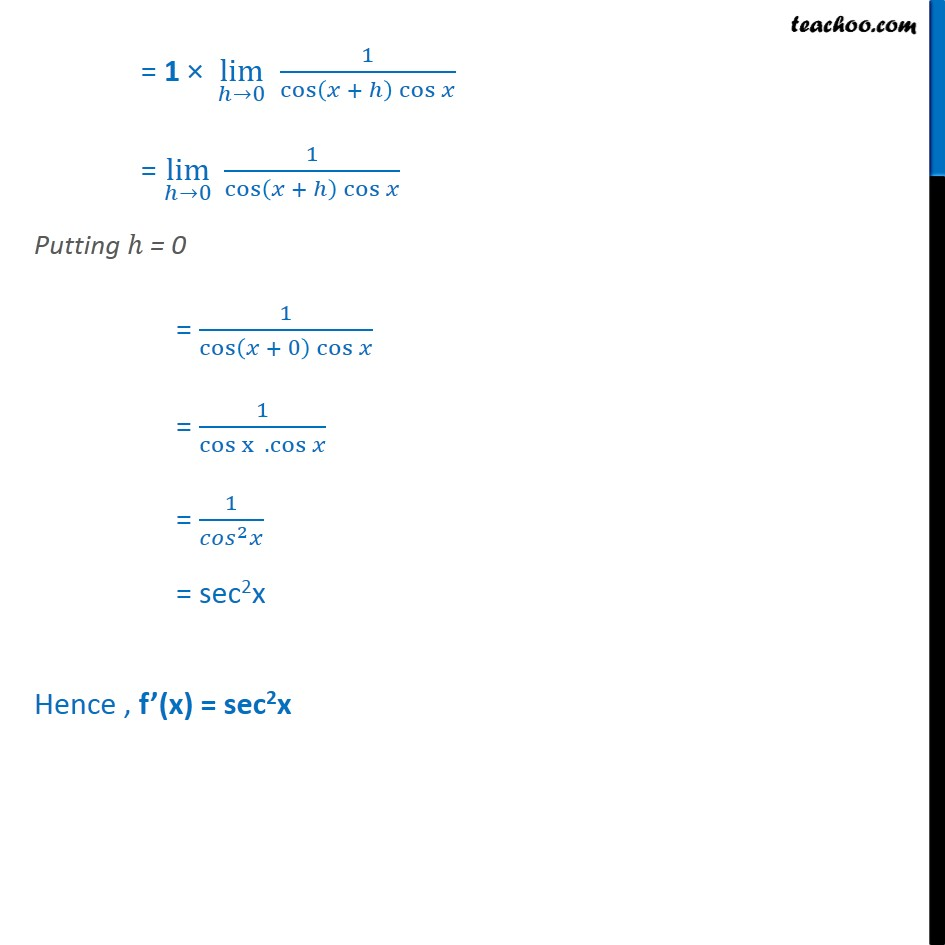1. Chapter 13 Class 11 Limits and Derivatives
2. Concept wise
3. Derivatives by formula - other trignometric

Transcript

Example 17 Compute the derivative tan x. Let f(x) = tan x We need to find f’ (x) We know that f’(x) = lim﷮ℎ→0﷯ f﷮ 𝑥 + ℎ﷯ − f (x)﷯﷮ℎ﷯ Here, f(x) = tan x So, f(x + ℎ) = tan (x + ℎ) Putting values f’ (x) = lim﷮ℎ→0﷯ tan﷮ 𝑥 + ℎ﷯ − tan﷮𝑥﷯﷯﷮ℎ﷯ f’ (x) = lim﷮ℎ→0﷯ 1﷮ℎ﷯ ( tan (x + h) – tan x) = lim﷮ℎ→0﷯ 1﷮ℎ﷯ sin﷮ 𝑥 + ℎ﷯﷯﷮ cos﷮ 𝑥 + ℎ﷯﷯﷯ − sin﷮𝑥﷯﷮ cos﷮𝑥﷯﷯﷯ = lim﷮ℎ→0﷯ 1﷮ℎ﷯ cos x sin﷮ 𝑥 + ℎ﷯ − cos﷮ 𝑥 + ℎ﷯ sin﷮𝑥﷯﷯﷯﷮ cos﷮ 𝑥 + ℎ﷯ cos﷮𝑥﷯﷯﷯﷯ = lim﷮ℎ→0﷯ 1﷮ℎ﷯ 𝒔𝒊𝒏﷮ 𝒙 + 𝒉﷯ 𝒄𝒐𝒔﷮𝒙 − 𝒄𝒐𝒔﷮ 𝒙 + 𝒉﷯. 𝒔𝒊𝒏﷮𝒙﷯﷯﷯﷯﷮ cos﷮ 𝑥 + ℎ﷯ cos﷮𝑥﷯﷯﷯﷯ = lim﷮ℎ→0﷯ 1﷮ℎ﷯ 𝐬𝐢𝐧﷮ 𝒙 + 𝒉 ﷯ − 𝒙﷯﷯﷮ cos﷮ 𝑥 + ℎ﷯ cos﷮𝑥﷯﷯﷯﷯ = lim﷮ℎ→0﷯ 1﷮ℎ﷯ ( sin﷮ 𝑥 + ℎ − 𝑥 ﷯) ﷯﷮ cos﷮ 𝑥 + ℎ﷯ cos﷮𝑥﷯﷯﷯ = lim﷮ℎ→0﷯ 1﷮ℎ﷯ ( sin﷮ ℎ﷯) ﷯﷮ cos﷮ 𝑥 + ℎ﷯ cos﷮𝑥﷯﷯﷯ = lim﷮ℎ→0﷯ sin﷮ℎ﷯﷮ℎ﷯ 1﷮ cos﷮ 𝑥 + ℎ﷯ cos﷮𝑥﷯﷯﷯ = 𝐥𝐢𝐦﷮𝒉→𝟎﷯ 𝒔𝒊𝒏﷮𝒉﷯﷮𝒉﷯ × lim﷮ℎ→0﷯ 1﷮ cos﷮ 𝑥 + ℎ﷯ cos﷮𝑥﷯﷯﷯ = 1 × lim﷮ℎ→0﷯ 1﷮ cos﷮ 𝑥 + ℎ﷯ cos﷮𝑥﷯﷯﷯ = lim﷮ℎ→0﷯ 1﷮ cos﷮ 𝑥 + ℎ﷯ cos﷮𝑥﷯﷯﷯ Putting ℎ = 0 = 1﷮ cos﷮ 𝑥 + 0﷯ cos﷮𝑥﷯﷯﷯ = 1﷮ cos x﷮ . cos﷮𝑥﷯﷯﷯ = 1﷮ 𝑐𝑜𝑠﷮2﷯𝑥﷯ = sec2x Hence , f’(x) = sec2x

Derivatives by formula - other trignometric